### Home > PC > Chapter 9 > Lesson 9.1.3 > Problem9-38

9-38.
1. Solve each equation without your calculator. Homework Help ✎

1. 8 = 24x

2. 2 = 8x

3. 1 = ex

4. log4 2 = x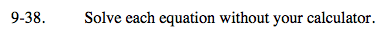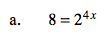23 = 24x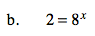See part (a) as an example.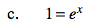Any number to what power is 1?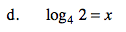4x = 2